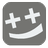Funny Addition
Elementary
RU English HU

We have an array of two positive integers. Add these two numbers together.

Input: A list of two elements. Each element is a positive integer.

Output: The sum of two numbers.

Example:

```checkio([5, 5]) == 10
checkio([7, 1]) == 8
checkio([5, 5]) == 10
```

How it is used: Just for fun.